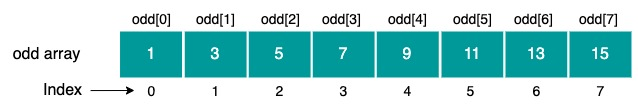# Java Program to Print All Elements of an Array

In this java program, we will learn about how to print the elements of array using for loop and by using Arrays.toString method.

Arrays is a data structure used to store multiple values of same type in a single variable, instead of using different variables for each value. Elements of the array can be accessed through their indexes.

Here is an example of an integer array containing eight elements. This is an array of odd numbers named "odd". Index of an array always starts from zero and goes up to n-1(n is size of array). In this case, as there are eight elements, the index is from 0 to 7.To understand this java program, you should have understanding of the following Java programming concepts:

## Java Program to Print an Array using For Loop

```public class PrintArrayLoop {
public static void main(String[] args) {
int[] array = {0, 1, 2, 3, 4, 5, 6, 7};
// Traverse array from index o to length-1
for(int i = 0; i < array.length; i++) {
System.out.print(array[i] + " ");
}
}
}
```
Output
```0 1 2 3 4 5 6 7
```

In the above java program, the for loop is used to iterate over the array from index 0 to length - 1 and print each array element using System.out.print() method.

## Java Program to Print an Array using Arrays.toString() Method

```import java.util.Arrays;

public class PrintArrayMethod {
public static void main(String[] args) {
int[] array = {0, 1, 2, 3, 4, 5, 6, 7};

System.out.println(Arrays.toString(array));
}
}
```
Output
```[0, 1, 2, 3, 4, 5, 6, 7]
```

In the above program, we are using Arrays.toString() method to print array elements.